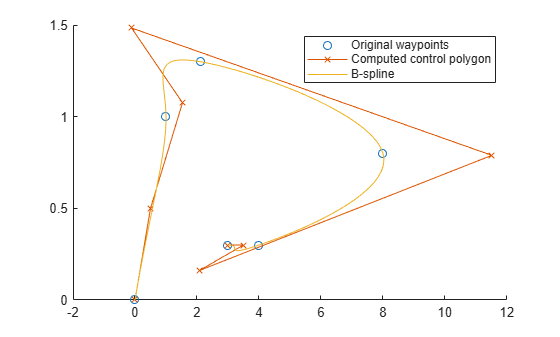# bsplinepolytraj

Generate polynomial trajectories using B-splines

Since R2019a

## Syntax

``[q,qd,qdd,pp] = bsplinepolytraj(controlPoints,tInterval,tSamples)``

## Description

example

````[q,qd,qdd,pp] = bsplinepolytraj(controlPoints,tInterval,tSamples)` generates a piecewise cubic B-spline trajectory that falls in the control polygon defined by `controlPoints`. The trajectory is uniformly sampled between the start and end times given in `tInterval`. The function returns the positions, velocities, and accelerations at the input time samples, `tSamples`. The function also returns the piecewise polynomial `pp` form of the polynomial trajectory with respect to time.```

## Examples

collapse all

Use the `bsplinepolytraj` function with a given set of 2-D xy control points. The B-spline uses these control points to create a trajectory inside the polygon. The start and end time for the trajectory are also given.

```cpts = [1 4 4 3 -2 0; 0 1 2 4 3 1]; tpts = [0 5];```

Compute the B-spline trajectory. The function outputs the trajectory positions (`q`), velocity (`qd`), acceleration (`qdd`), time vector (`tvec`), and polynomial coefficients (`pp`) of the polynomial that achieves the waypoints using the control points.

```tvec = 0:0.01:5; [q, qd, qdd, pp] = bsplinepolytraj(cpts,tpts,tvec);```

Plot the results. Show the control points and the resulting trajectory inside them.

```figure plot(cpts(1,:),cpts(2,:),'xb-') hold all plot(q(1,:), q(2,:)) xlabel('X') ylabel('Y') hold off```Plot the position of each element of the B-spline trajectory. These trajectories are cubic piecewise polynomials parameterized in time.

```figure plot(tvec,q) hold all plot([0:length(cpts)-1],cpts,'x') xlabel('t') ylabel('Position Value') legend('X-positions','Y-positions') hold off```Create waypoints to interpolate with a B-Spline.

```wpts1 = [0 1 2.1 8 4 3]; wpts2 = [0 1 1.3 .8 .3 .3]; wpts = [wpts1; wpts2]; L = length(wpts) - 1;```

Form matrices used to compute interior points of control polygon

```r = zeros(L+1, size(wpts,1)); A = eye(L+1); for i= 1:(L-1) A(i+1,(i):(i+2)) = [1 4 1]; r(i+1,:) = 6*wpts(:,i+1)'; end```

Override end points and choose `r0` and `rL`.

```A(2,1:3) = [3/2 7/2 1]; A(L,(L-1):(L+1)) = [1 7/2 3/2]; r(1,:) = (wpts(:,1) + (wpts(:,2) - wpts(:,1))/2)'; r(end,:) = (wpts(:,end-1) + (wpts(:,end) - wpts(:,end-1))/2)'; dInterior = (A\r)';```

Construct a complete control polygon and use `bsplinepolytraj` to compute a polynomial with the new control points

```cpts = [wpts(:,1) dInterior wpts(:,end)]; t = 0:0.01:1; q = bsplinepolytraj(cpts, [0 1], t);```

Plot the results. Show the original waypoints, the computed polygon, and the interpolated B-spline.

```figure; hold all plot(wpts1, wpts2, 'o'); plot(cpts(1,:), cpts(2,:), 'x-'); plot(q(1,:), q(2,:)); legend('Original waypoints', 'Computed control polygon', 'B-spline');```Farin, Section 9.1

## Input Arguments

collapse all

Points for control polygon of B-spline trajectory, specified as an n-by-p matrix, where n is the dimension of the trajectory and p is the number of control points.

Example: `[1 4 4 3 -2 0; 0 1 2 4 3 1]`

Data Types: `single` | `double`

Start and end times for the trajectory, specified as a two-element vector.

Example: `[0 10]`

Data Types: `single` | `double`

Time samples for the trajectory, specified as a vector. The output position, `q`, velocity, `qd`, and accelerations, `qdd`, are sampled at these time intervals.

Example: `0:0.01:10`

Data Types: `single` | `double`

## Output Arguments

collapse all

Positions of the trajectory at the given time samples in `tSamples`, returned as a vector.

Data Types: `single` | `double`

Velocities of the trajectory at the given time samples in `tSamples`, returned as a vector.

Data Types: `single` | `double`

Accelerations of the trajectory at the given time samples in `tSamples`, returned as a vector.

Data Types: `single` | `double`

Piecewise-polynomial, returned as a structure that defines the polynomial for each section of the piecewise trajectory. You can build your own piecewise polynomials using `mkpp`, or evaluate the polynomial at specified times using `ppval`. The structure contains the fields:

• `form`: `'pp'`.

• `breaks`: p-element vector of times when the piecewise trajectory changes forms. p is the number of waypoints.

• `coefs`: n(p–1)-by-`order` matrix for the coefficients for the polynomials. n(p–1) is the dimension of the trajectory times the number of `pieces`. Each set of n rows defines the coefficients for the polynomial that described each variable trajectory.

• `pieces`: p–1. The number of breaks minus 1.

• `order`: Degree of the polynomial + 1. For example, cubic polynomials have an order of 4.

• `dim`: n. The dimension of the control point positions.

 Farin, Gerald E. Curves and Surfaces for Computer Aided Geometric Design: A Practical Guide. San Diego, CA: Academic Press, 1993.